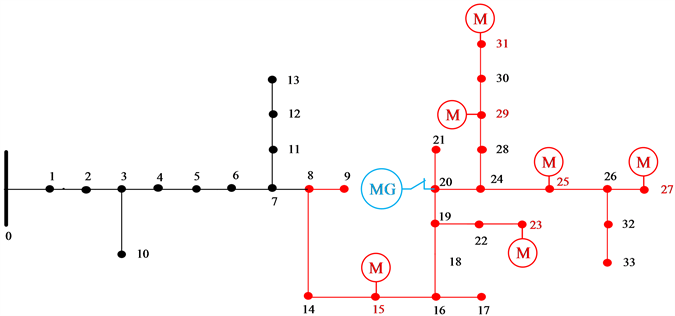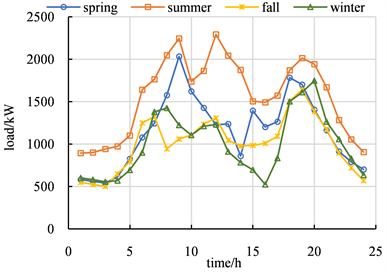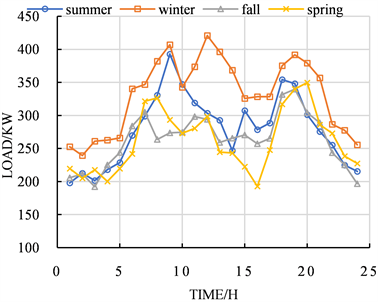﻿ 基于分布式电源重要度的微电网支撑能力评价指标体系研究

# 基于分布式电源重要度的微电网支撑能力评价指标体系研究Research on Evaluation Index System of Microgrid Support Capability Based on Importance of Distributed Power

Abstract: In recent years, large-scale blackouts in power grid caused by natural disasters such as typhoons and earthquakes have occurred frequently. Considering the controllable source and load in the micro-grid have flexible power control capability, which can support the grid voltage and power. So this paper proposes a new idea: using the micro-grid to support secure power grid by coordinating active and reactive power. Firstly, it studies the active and reactive power characteristics of various controllable source loads and their influence on the system voltage; secondly, establishing the evaluation index of capacity support capability, by considering the power support capability and support time of the micro-grid to the guaranteed power grid; thirdly, proposing the micro-grid planning idea based on active and reactive power coordination, and the micro-grid planning method for improving the micro-grid support capacity; fourthly, establishing the micro-grid optimization model. Considering the constraints of voltage, power flow and power output, realizing the optimal planning of controllable source and load in the micro-grid by using XX algorithm. Finally, a numerical case is given to verify the effectiveness of the proposed micro-grid planning method.

1. 引言

2. 考虑微电网ESC的DG优化配置模型

2.1. 微电网应急支撑能力评价指标体系

(1) 经济性指标

${F}_{2}=\mathrm{max}\left\{{C}_{C}+{C}_{OM}\right\}$ (1)

${C}_{C}={P}_{pv}{C}_{pv}^{in}+{P}_{wt}{C}_{wt}^{in}+{P}_{mt}{C}_{mt}^{in}+{P}_{es}{C}_{es}^{in}$ (2)

${C}_{OM}=\underset{n=1}{\overset{N}{\sum }}\left[{P}_{wt}{C}_{wt}^{op}+{P}_{pv}{C}_{pv}^{op}+{P}_{es}{C}_{es}^{op}+{P}_{mt}{C}_{mt}^{op}\right]$ (3)

(2) 性能指标

1) 最大负荷缺电率

${f}_{lps}=\underset{s=1}{\overset{4}{\sum }}{T}_{s}\underset{t=1}{\overset{T}{\sum }}\left[\frac{\left({\alpha }_{t}{P}_{wt}+{\beta }_{t}{P}_{pv}+{P}_{mt}+{P}_{es}-{P}_{t,s}^{M}\right)+\left({P}_{t,s}^{L,D}-{P}_{t,s}^{L}\right)}{{P}_{t,s}^{L}}\right]$ (5)

2) 微电网等值电源可靠性

${\lambda }_{EVE}={\int }_{0}^{T}\left[{P}_{s}\left(t\right)-{P}_{L}\left(t\right)\right]\text{d}t$ (6)

${\lambda }_{EVE}$ 受到微电网内一次动力分布、DG的配置、控制策略、功率输出特性和故障停运，以及负荷特性等因素影响。

3) 微电网间歇性DG可靠性

${\lambda }_{AIDG}=\frac{{\int }_{0}^{T}P\left(t\right)\text{d}t}{{P}_{DGN}T}$ (7)

${\lambda }_{AIP}=\mathrm{Pr}\left\{{P}_{DG}=0\right\}$ (8)

${\lambda }_{AIP}$ 描述了DG出力间断的程度，反映了孤岛运行时DG对微网内负荷可靠供电的能力，也是影响SAIFI和CAIFI的重要因素。

4) 储能装置持续出力等效时间

${\lambda }_{ETSSO}=\frac{{E}_{s}}{\sum {P}_{DGN}}$ (9)

${\lambda }_{ETSSO}$ 相当于混合发电系统所配置的储能装置按DG的额定功率释放电力的等效工作时间，是影响微网孤岛状态下持续供电能力的重要因素，也是储能装置优化配置的重要指标。

5) 微型燃气轮机(或内燃机)容量占比

${\lambda }_{mt}=\frac{{P}_{mt}}{{P}_{wt}+{P}_{pv}+{P}_{mt}+{E}_{s}}$ (10)

2.2. 约束条件

(1) DG装机容量约束

${N}_{wt}\le \left[\frac{L}{8d}+1\right]\cdot \left[\frac{W}{4d}+1\right]$ (11)

${N}_{pv}\le \left[\frac{S}{{S}_{pv}}\right]$ (12)

(2) 储能充放电约束

$\left\{\begin{array}{l}\frac{{G}_{t}^{es,cap}-{G}_{t-1}^{es,cap}+{P}_{t,s}^{es-dis}}{\eta }-\eta {P}_{t,s}^{es-ch}=0,t>2\\ \frac{{G}_{t=1}^{es,cap}+{P}_{t,s}^{es-dis}}{\eta }-\eta {P}_{t=1}^{es-ch}={G}_{t=0}^{es,cap}\\ {G}_{\mathrm{min}}^{es,cap}\le {G}_{t}^{es,cap}\le {G}_{\mathrm{max}}^{es,cap}\end{array}$ (13)

(3) 功率平衡约束

$\left\{\begin{array}{l}{P}_{t}^{M}={P}_{t}^{mt}+{P}_{t}^{es}+\underset{r\in {N}_{r}}{\sum }{R}_{t}^{r}+{P}_{t}^{L,D}-{P}_{t}^{MG-DN}\\ {P}_{h}^{MG-DN}>0\end{array}$ (14)

(4) 微电网与保底电网功率交换约束

${P}_{h}^{MG-DN}\le {P}_{h,\mathrm{max}}^{MG-DN}$ (15)

(5) 并网节点电压约束

${U}_{i,\mathrm{min}}\le {U}_{i}\le {U}_{i,\mathrm{max}}$ (16)

2.3. 求解方法

${{f}^{\prime }}_{lps}=\frac{\mathrm{max}{f}_{lps}-{f}_{lps}}{\mathrm{max}{f}_{lps}-\mathrm{min}{f}_{lps}}$ (17)

${{C}^{\prime }}_{A}=\frac{{C}_{A}-\mathrm{min}{C}_{A}}{\mathrm{max}{C}_{A}-\mathrm{min}{C}_{A}}$ (18)

$U={\left({u}_{ji}\right)}_{n×n}$ (19)

${W}_{j}={\left(\underset{i=1}{\overset{n}{\prod }}{u}_{ij}\right)}^{1/n}$ (20)

${p}_{i}=\frac{fitness\left({x}_{i}\right)}{\underset{i=1}{\overset{NP}{\sum }}fitness\left({x}_{i}\right)}$ (21)

Step 1：随机产生初始种群，数目为Nw，每个个体表示为染色体的基因编码；

Step 2：用轮盘赌策略确定个体的适应度为fitness (xi)，并判断是否符合优化准则；若符合则输出最优解，若不符合，则转向Step 3；

Step 3：依据适应度选择再生个体，适应度高的个体被选中的概率高，适应度低的个体被淘汰；

Step 4：按照单点交叉方法生成新的个体；

Step 5：按照单点变异方法生成新的个体；

Step 6：由交叉和变异产生新一代的种群，回到Step 2。

3. 算例分析Figure 1. Distribution network 34 node structure diagramFigure 2. Guaranteed grid load data in a certain areaFigure 3. Microgrid load data in a certain areaTable 1. The parameters of the DGTable 2. Weight of each indicatorTable 3. Calculation results for each sceneTable 4. Calculation results for each scene

4. 结论

 郇嘉嘉, 韦斌, 隋宇, 等. 一种城市防风抗灾保底电网的多目标规划方法[J]. 电网技术, 2018(3): 927-932.

 董飞飞, 刘涤尘, 吴军, 等. 基于改进BBO优化算法和电网生存性的核心骨干网架构建[J]. 中国电机工程学报, 2014, 34(16): 2659-2667.

 Li, J., Ma, X.Y., Liu, C.C., et al. (2014) Distribution System Restoration with Microgrids Using Spanning Tree Search. IEEE Transactions on Power Systems, 29, 3021-3029.
https://doi.org/10.1109/TPWRS.2014.2312424

 唐忠, 田晨, 资容涛. 多微电网互联系统的储能容量配置[J]. 电测与仪表, 2017, 56(4): 98-111.

 杨新法, 苏剑, 吕志鹏, 等. 微电网技术综述[J]. 中国电机工程学报, 2014, 34(1): 57-70.

 Bahramirad, S., Reder, W. and Khodaei, A. (2012) Reliability-Constrained Optimal Sizing of Energy Storage System in a Microgrid. IEEE Transactions on Smart Grid, 3, 2056-2062.
https://doi.org/10.1109/TSG.2012.2217991

 殷桂梁, 李相男, 郭磊, 等. 混合储能系统在风光互补微电网中的应用[J]. 电力系统及其自动化学报, 2015, 27(1): 49-53.

 谭兴国, 王辉, 张黎, 等. 微电网复合储能多目标优化配置方法及评价指标[J]. 电力系统自动化, 2014, 38(8): 7-14.

 杨昆, 周晓健, 夏能弘, 等. 考虑微电网接入下的配电网优化规划[J]. 电测与仪表, 2019, 56(2): 58-64 + 75.

 Jiaoyang L, Li G, Shuqiang Y, et al. (2018) Optimal Sizing for Multi PV-ESS Microgrids in Distribution Network. Power System Technology.

 Zeng, Z., Zhao, R. and Yang, H. (2013) Micro-Sources Design of an Intelligent Building Integrated with Micro-Grid. Energy and Buildings, 57, 261-267.
https://doi.org/10.1016/j.enbuild.2012.11.018

 王蓓蓓, 赵盛楠, 刘小聪, 等. 面向可再生能源消纳的智能用电关键技术分析与思考[J]. 电网技术, 2016, 40(12): 295-304.

 王瑞琪, 李珂, 张承慧. 基于混沌多目标遗传算法的微网系统容量优化[J]. 电力系统保护与控制, 2011, 39(22): 16-22.

 Zhou, Y. and Li, H. (2014) Analysis and Suppression of Leakage Current in Cascaded-Multilevel-Inverter-Based PV Systems. IEEE Transactions on Power Electronics, 29, 5265-5277.
https://doi.org/10.1109/TPEL.2013.2289939

 刘万琨. 风能与风力发电技术[M]. 北京: 化学工业出版社, 2006.

 焦立新. 评价指标标准化处理方法的探讨[J]. 安徽科技学院学报, 1999, 13(3): 7-10.

 胡晓通, 刘天琪, 何川, 等. 计及蓄电池损耗特性的微网多目标优化运行[J]. 中国电机工程学报, 2016, 36(10): 2674-2681.

 胡林静, 刘凯, 杨明文. 基于粒子群遗传算法的光伏MPPT控制研究[J]. 电测与仪表, 2019, 56(14): 23-27.

 刘娇扬, 郭力, 杨书强, 等. 配电网中多光储微网系统的优化配置方法[J]. 电网技术, 2018, 42(9): 2806-2815.

 徐林, 阮新波, 张步涵, 等. 风光蓄互补发电系统容量的改进优化配置方法[J]. 中国电机工程学报, 2012, 32(25): 88-98.

Top